SE301: Introduction to Numerical Methods Summer 033     Sections 5 and 6 Computer Assignment   Instructor:  Dr. Samir Al-Amer     General Instructions: The grades of the best five computer assignments are used in computing the computer Assignment grade. The grade for each computer assignment is as follows: 2:   The program is well-written and the results are correct 1:   A series attempt to solve the problem was done but the program is not       complete or the answer is not correct. 0:  The assignment was not submitted or submitted too late or no series         attempt to solve the problem was done. Write your name and ID # at the top of your program. Use MATLAB to solve the computer assignments. Other programming languages may be allowed but prior permission of the instructor is required. Late submissions will not be accepted. Submit your CHW using the WebCT course account.   Computer Assignment # 1                                    Due to :_____________          The Nth order Taylor series expansion of f(x) = sin(x) is given by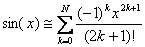Write a program that computes sin(0.2) using N = 1, 2, 3, 4 and 5. Compute and print the percentage relative error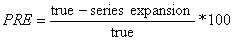Use the built-in sine function to evaluate the true value. Display the result in a tabulated form.   ______________________________________________________________________________         Computer Assignment # 2                                    Due to :_____________   The volume V of a liquid in a spherical tank of radius  r is related to the depth h  of the liquid is given by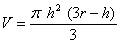.    Given h = 1m  and V = 0.5m3 Assuming the initial interval [0,2], Write a program that uses Bisection method to determine h with four-decimal-digit accuracy. Your program should display the estimated level.  ______________________________________________________________________________         Computer Assignment # 3                                    Due to :_____________   The trajectory of a ball thrown by a player is defined in terms of its (x,y)-coordinates. The trajectory is modeled by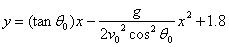where g = 9.81 and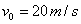. Write a program that implements the Secant method to determine the initial angle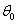with four-decimal-digit accuracy so that the ball hit a target with coordinate (40,1). Use 30 and 40 degrees as initial guesses.    ______________________________________________________________________________         Computer Assignment # 4                                    Due to :_____________   The node-voltage law was applied to an electrical circuit and the following equations were obtained.Write a program that implements the Naive Gaussian elimination and use it to solve for the unknowns V1, V2 and V3 and display their values.           Computer Assignment # 5                                    Due to :_____________   The upward velocity of a rocket can be computed as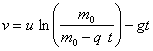where u    :   velocity at which burned fuel exit the rocket (u = 2000m/s)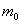:   initial mass of the rocket  (= 150000 kg) q    :  fuel consumption rate  (q = 2600 kg/s) g    :  gravitational constant   (g = 9.81) t     :  time   Write a program that implements the Romberg method of order O(h8) to compute the height the rocket reaches after 30 seconds. Your program should display the Romberg table.     ______________________________________________________________________________         Computer Assignment # 6                                    Due to :_____________   A mathematical model for the area A  (in  cm2) that a colony of bacteria occupies is given by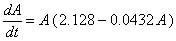Write a program that uses the Fourth order Runge-Kutta method to compute the area over the interval [0, 5]. Assume  the initial area is 0.2 cm2 and use h = 0.05. Your program should display the area after 5 time units and a graph to show the area during the studied interval is also needed.